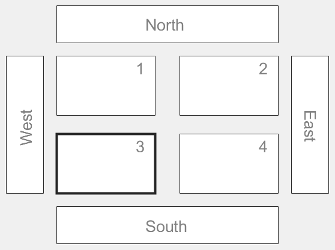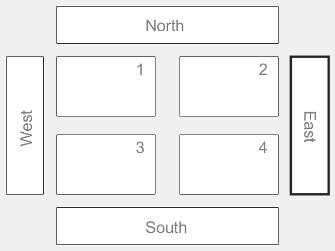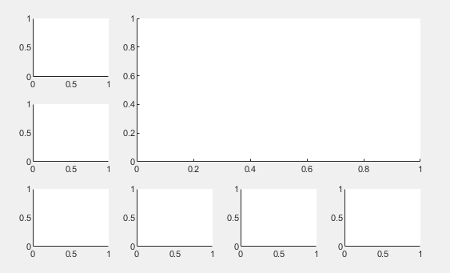# nexttile

## 语法

``nexttile``
``nexttile(span)``
``nexttile(tilelocation)``
``nexttile(tilelocation,span)``
``nexttile(t,___)``
``ax = nexttile(___)``

## 说明

``nexttile` 创建一个坐标区对象，再将其放入当前图窗中的分块图布局的下一个空图块中。如果当前图窗中没有布局，则 `nexttile` 会创建一个新布局并使用 `'flow'` 图块排列进行配置。生成的坐标区对象是当前坐标区，因此下一个绘图命令可以在其中进行绘制。`

``nexttile(span)` 创建一个占据布局中心网格多行或多列的坐标区对象。指定 `span` 作为 `[r c]` 形式的向量。坐标区占据 `r` 行×`c` 列的图块。坐标区的左上角位于网格中第一个空的 `r`×`c` 区域的左上角。`

````nexttile(tilelocation)` 将当前坐标区指定为 `tilelocation` 指定的图块中的坐标区或独立可视化。通常，当您要修改现有坐标区或独立可视化时，此语法非常有用。然而，在某些情况下，`nexttile` 会创建一个新坐标区对象： 当指定的图块为空时，`nexttile` 会在该图块中创建坐标区对象。如果指定的图块包含坐标区或独立可视化对象的一部分但不包含其左上角，`nexttile` 将替换现有对象。例如，如果 `tilelocation` 引用占据多个图块的坐标区对象中间的图块，`nexttile` 会在您指定的图块中用新坐标区对象替换现有坐标区对象。 ```

````nexttile(tilelocation,span)` 创建一个占据多行或多列的坐标区对象，从 `tilelocation` 指定的图块开始。如果您指定的图块中有坐标区或独立可视化，`nexttile` 会将该对象设为当前坐标区或替换它： 如果现有坐标区或独立可视化对象占据与 `tilelocation` 和 `span` 参数指定的相同的图块集，则 `nexttile` 会将该对象设为当前坐标区。如果现有坐标区或独立可视化对象占据的图块集不同于 `tilelocation` 和 `span` 参数指定的图块集，则 `nexttile` 会使用新的 `tilelocation` 和 `span` 值用新的坐标区对象替换现有对象。 ```

``nexttile(t,___)` 对 `t` 指定的分块图布局进行操作。请在所有其他输入参数之前指定 `t`。如果您使用多个布局，或布局位于面板或选项卡（而不是图窗）中，则此语法非常有用。`

``ax = nexttile(___)` 返回坐标区对象。使用 `ax` 对坐标区设置属性。您也可以将 `ax` 作为输入参数传递给对坐标区对象执行操作的其他图形函数。例如，您可以调用 `colormap` 或 `colororder` 函数来更改坐标区的颜色方案。`

## 示例

```x = linspace(0,30); y1 = sin(x/2); y2 = sin(x/3); y3 = sin(x/4); % Plot into first tile three times nexttile plot(x,y1)``````nexttile plot(x,y2)``````nexttile plot(x,y3)``````nexttile plot(x,y1) hold on plot(x,y2) plot(x,y3) hold off``````tiledlayout(2,2); [X,Y,Z] = peaks(20); % Tile 1 nexttile surf(X,Y,Z) % Tile 2 nexttile contour(X,Y,Z) % Tile 3 nexttile imagesc(Z) % Tile 4 nexttile plot3(X,Y,Z)``````t = tiledlayout(2,1); % First tile ax1 = nexttile; plot([1 2 3 4 5],[11 6 10 4 18]); ax1.XColor = [1 0 0]; ax1.YColor = [1 0 0]; % Second tile ax2 = nexttile; plot([1 2 3 4 5],[5 1 12 9 2],'o'); ax2.XColor = [1 0 0]; ax2.YColor = [1 0 0];````scores``strikes` 定义为包含四场保龄球联赛数据的向量。然后创建一个 `3`×`3` 分块图布局，并显示五个条形图，其中显示每个团队的击球次数。

```scores = [444 460 380 388 389 387 366 500 467 460 365 451 611 426 495 548 412 452 471 402]; strikes = [9 6 5 7 5 6 4 8 10 7 4 7 16 9 9 10 9 8 8 9]; t = tiledlayout(3,3); % Team 1 nexttile bar([1 2 3 4],strikes(:,1)) title('Team 1 Strikes') % Team 2 nexttile bar([1 2 3 4],strikes(:,2)) title('Team 2 Strikes') % Team 3 nexttile bar([1 2 3 4],strikes(:,3)) title('Team 3 Strikes') % Team 4 nexttile bar([1 2 3 4],strikes(:,4)) title('Team 4 Strikes') % Team 5 nexttile(7) bar([1 2 3 4],strikes(:,5)) title('Team 5 Strikes')``````nexttile(5,[2 2]); plot([1 2 3 4],scores,'-.') labels = {'Team 1','Team 2','Team 3','Team 4','Team 5'}; legend(labels,'Location','northwest') % Configure ticks and axis labels xticks([1 2 3 4]) xlabel('Game') ylabel('Score') % Add layout title title(t,'April Bowling League Data')``````tiledlayout(1,2) % Display geographic plot nexttile geoplot([47.62 61.20],[-122.33 -149.90],'g-*') % Display polar plot nexttile theta = pi/4:pi/4:2*pi; rho = [19 6 12 18 16 11 15 15]; polarscatter(theta,rho)````nexttile` 输出参数的一个有用的用途体现在您想调整前一个图块中的内容时。例如，您可能决定要重新配置先前绘图中使用的颜色图。

```tiledlayout(2,2); [X,Y,Z] = peaks(20); % Tile 1 nexttile surf(X,Y,Z) % Tile 2 nexttile contour(X,Y,Z) % Tile 3 nexttile imagesc(Z) % Tile 4 nexttile plot3(X,Y,Z)``````ax = nexttile(3); colormap(ax,cool)``````t = tiledlayout(2,3); [X,Y,Z] = peaks; % Tile 1 nexttile contour(X,Y,Z) % Span across two rows and columns nexttile([2 2]) contourf(X,Y,Z) % Last tile nexttile imagesc(Z)``````ax = nexttile(2); colormap(ax,hot)``````load patients tbl = table(Diastolic,Smoker,Systolic,Height,Weight,SelfAssessedHealthStatus); tiledlayout(2,2) % Scatter plot nexttile scatter(tbl.Height,tbl.Weight) % Heatmap nexttile heatmap(tbl,'Smoker','SelfAssessedHealthStatus','Title','Smoker''s Health'); % Stacked plot nexttile([1 2]) stackedplot(tbl,{'Systolic','Diastolic'});``````nexttile(1) scatterhistogram(tbl,'Height','Weight');``````Z1 = peaks; Z2 = membrane; tiledlayout(2,1); nexttile contourf(Z1) nexttile contourf(Z2)``````cb = colorbar; cb.Layout.Tile = 'east';``````p = uipanel('Position',[.1 .2 .8 .6]); t = tiledlayout(p,2,1); % Tile 1 nexttile(t) stem(1:13) % Tile 2 nexttile(t) bar([10 22 31 43 52])``````t = tiledlayout('flow'); nexttile plot(rand(1,10)); nexttile plot(rand(1,10)); nexttile plot(rand(1,10));``````gax = geoaxes(t); gax.Layout.Tile = 4; gax.Layout.TileSpan = [2 3];``````geoplot(gax,[47.62 61.20],[-122.33 -149.90],'g-*') gax.MapCenter = [47.62 -122.33]; gax.ZoomLevel = 2;```## 输入参数

`tilelocation`说明示例

```tiledlayout(2,2) nexttile(3)````'north'``'south'``'east'``'west'`网格外层的一个图块。

```tiledlayout(2,2) nexttile('east')```